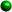Homework Tips for GraphingDetermining if Ordered Pairs are Solutions to EquationsIf given an ordered pair, substitute in the equation and if you get a true statement the pair is a solution to the equation.To complete an ordered pair so that it is a solution to an equation, sustitute the x value or y value into the equation and solve for the other variable. Plotting a PointThe rectangular coordinate system is made up of two number lines, one horizontal called the x axis and one vertical called the y axis. A point consists of an ordered pair. The first coordionate is always the x value and the second coordinate is always the y value.In order to plot a point, start at the origin(0,0). move horizontally (right or left depending on the sign of x) the number of units of the x coordinate and go vertically (up of down depending on the y coordinate). Now plot your point. It takes TWO coordinates to plot a point. Graphing a Straight LineDraw a "t" table for the values of x and y.We want to find three points on the line.First, make x = 0 and find the y value when x = 0 by substituting in the equation.Next, make y = 0 and substitute in the equation to find the value of x when y = 0.Connect the two points and draw the line.If we only use these two point we are graphing using the intercept method. The x intercept is where the line touches the x axis (y=0) and the y intercept is where the line touches the y axis (x = 0). We should use a third point to make sure we have not made an error. Now, make x or y anything you wish and substitute back into the equation to get your third point.